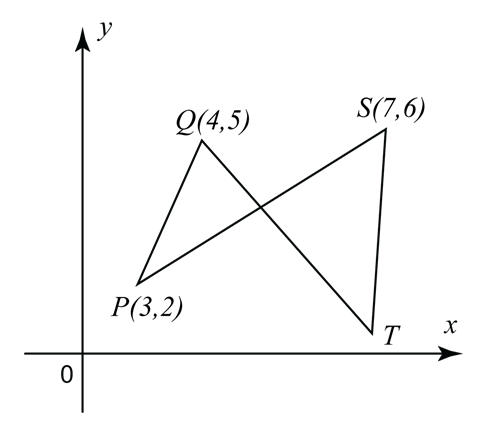# 6.8.2 Coordinate Geometry Long Questions (Question 3 & 4)

Question 3:In the diagram, PRS and QRT are straight lines. Given is the midpoint of PS and
QR : RT = 1 : 3, Find
(a) the coordinates of R,
(b) the coordinates of T,
(c) the coordinates of the point of intersection between lines PQ and ST produced.

Solution:
(a)
Given R is the midpoint of PS.

(b)

(c)

Substitute (1) into (2),
3x – 7 = –5x + 41
8x = 48
x = 6

From (1),
y = 3(6) – 7 = 11

The coordinates of the point of intersection between lines PQ and ST = (6, 11).

Question 4:The diagram shows a triangle LMN where L is on the y-axis. The equation of the straight line LKN and MK are 2y – 3x + 6 = 0 and 3y + x– 13 = 0 respectively. Find
(a) the coordinates of K
(b) the ratio LK:KN

Solution:
(a)
2y – 3x + 6 = 0 —-(1)
3y + x – 13 = 0 —-(2)
x = 13 – 3y —-(3)

Substitute equation (3) into (1),
2y – 3 (13 – 3y) + 6 = 0
2y – 39 + 9y + 6 = 0
11y = 33
y = 3
Substitute y = 3 into equation (3),
x = 13 – 3 (3)
x = 4
Coordinates of K = (4, 3).

(b)
Given equation of LKN is 2y – 3x + 6 = 0
At y – axis, x = 0,
x coordinates of point L = 0.